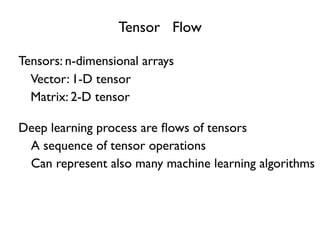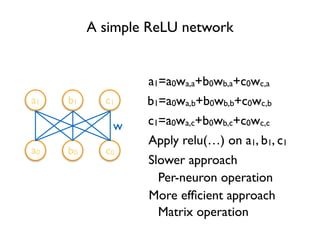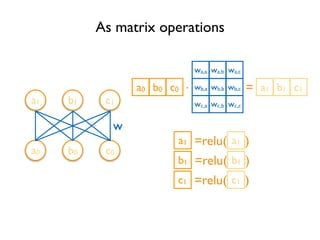Successfully reported this slideshow.

290

Share×
1 of 44
1 of 44

290

Share

#### Description

TensorFlow Tutorial given by Dr. Chung-Cheng Chiu at Google Brain on Dec. 29, 2015

#### Transcript

1. 1. Tensor Flow Tensors: n-dimensional arrays A sequence of tensor operations Deep learning process are ﬂows of tensors Vector: 1-D tensor Matrix: 2-D tensor Can represent also many machine learning algorithms
2. 2. A simple ReLU network a1 b1 c1 a0 b0 c0 w a1=a0wa,a+b0wb,a+c0wc,a b1=a0wa,b+b0wb,b+c0wc,b c1=a0wa,c+b0wb,c+c0wc,c Apply relu(…) on a1, b1, c1 Slower approach Per-neuron operation More efﬁcient approach Matrix operation
3. 3. As matrix operations a0 a1 b1 c1 a0 b0 c0 w . = =relu( ) b0 c0 a1 b1 c1 a1a1 =relu( )b1b1 =relu( )c1c1 wa,a wb,a wc,a wa,b wb,b wc,b wa,c wb,c wc,c
4. 4. With TensorFlow a1 b1 c1 a0 b0 c0 w out = tf.nn.relu(y) y = tf.matmul(x, w) x w a0 . =b0 c0 wa,a wb,a wc,a wa,b wb,b wc,b wa,c wb,c wc,c a1 b1 c1 =relu( )a1a1 =relu( )b1b1 =relu( )c1c1 import tensorﬂow as tf
5. 5. Deﬁne Tensors xa,a xb,a xc,a xa,b xb,b xc,b xa,c xb,c xc,c w Variable(<initial-value>, name=<optional-name>) w = tf.Variable(tf.random_normal([3, 3]), name='w') import tensorﬂow as tf y = tf.matmul(x, w) relu_out = tf.nn.relu(y) Variable stores the state of current execution Others are operations
6. 6. TensorFlow Code so far deﬁnes a data ﬂow graph MatMul ReLU Variable x w = tf.Variable(tf.random_normal([3, 3]), name='w') import tensorﬂow as tf y = tf.matmul(x, w) relu_out = tf.nn.relu(y) Each variable corresponds to a node in the graph, not the result Can be confusing at the beginning
7. 7. TensorFlow Code so far deﬁnes a data ﬂow graph Needs to specify how we want to execute the graph MatMul ReLU Variable x Session Manage resource for graph execution w = tf.Variable(tf.random_normal([3, 3]), name='w') sess = tf.Session() y = tf.matmul(x, w) relu_out = tf.nn.relu(y) import tensorﬂow as tf result = sess.run(relu_out)
8. 8. Graph Fetch Retrieve content from a node w = tf.Variable(tf.random_normal([3, 3]), name='w') sess = tf.Session() y = tf.matmul(x, w) relu_out = tf.nn.relu(y) import tensorﬂow as tf print sess.run(relu_out) MatMul ReLU Variable x Fetch We have assembled the pipes Fetch the liquid
9. 9. Graph sess = tf.Session() y = tf.matmul(x, w) relu_out = tf.nn.relu(y) import tensorﬂow as tf print sess.run(relu_out) sess.run(tf.initialize_all_variables()) w = tf.Variable(tf.random_normal([3, 3]), name='w') InitializeVariable Variable is an empty node MatMul ReLU Variable x Fetch Fill in the content of a Variable node
10. 10. Graph sess = tf.Session() y = tf.matmul(x, w) relu_out = tf.nn.relu(y) import tensorﬂow as tf print sess.run(relu_out) sess.run(tf.initialize_all_variables()) w = tf.Variable(tf.random_normal([3, 3]), name='w') x = tf.placeholder("ﬂoat", [1, 3]) Placeholder How about x? MatMul ReLU Variable x Fetch placeholder(<data type>, shape=<optional-shape>, name=<optional-name>) Its content will be fed
11. 11. Graph import numpy as np import tensorﬂow as tf sess = tf.Session() x = tf.placeholder("ﬂoat", [1, 3]) w = tf.Variable(tf.random_normal([3, 3]), name='w') y = tf.matmul(x, w) relu_out = tf.nn.relu(y) sess.run(tf.initialize_all_variables()) print sess.run(relu_out, feed_dict={x:np.array([[1.0, 2.0, 3.0]])}) Feed MatMul ReLU Variable x FetchPump liquid into the pipe Feed
12. 12. Session management Needs to release resource after use sess.close() Common usage with tf.Session() as sess: … Interactive sess = InteractiveSession()
13. 13. Prediction import numpy as np import tensorﬂow as tf with tf.Session() as sess: x = tf.placeholder("ﬂoat", [1, 3]) w = tf.Variable(tf.random_normal([3, 3]), name='w') relu_out = tf.nn.relu(tf.matmul(x, w)) softmax = tf.nn.softmax(relu_out) sess.run(tf.initialize_all_variables()) print sess.run(softmax, feed_dict={x:np.array([[1.0, 2.0, 3.0]])}) Softmax Make predictions for n targets that sum to 1
14. 14. Prediction Difference import numpy as np import tensorﬂow as tf with tf.Session() as sess: x = tf.placeholder("ﬂoat", [1, 3]) w = tf.Variable(tf.random_normal([3, 3]), name='w') relu_out = tf.nn.relu(tf.matmul(x, w)) softmax = tf.nn.softmax(relu_out) sess.run(tf.initialize_all_variables()) answer = np.array([[0.0, 1.0, 0.0]]) print answer - sess.run(softmax, feed_dict={x:np.array([[1.0, 2.0, 3.0]])})
15. 15. Learn parameters: Loss Deﬁne loss function Loss function for softmax softmax_cross_entropy_with_logits( logits, labels, name=<optional-name>) labels = tf.placeholder("ﬂoat", [1, 3]) cross_entropy = tf.nn.softmax_cross_entropy_with_logits( relu_out, labels, name='xentropy')
16. 16. Learn parameters: Optimization Gradient descent class GradientDescentOptimizer GradientDescentOptimizer(learning rate) labels = tf.placeholder("ﬂoat", [1, 3]) cross_entropy = tf.nn.softmax_cross_entropy_with_logits( relu_out, labels, name='xentropy') optimizer = tf.train.GradientDescentOptimizer(0.1) train_op = optimizer.minimize(cross_entropy) sess.run(train_op, feed_dict= {x:np.array([[1.0, 2.0, 3.0]]), labels:answer}) learning rate = 0.1
17. 17. Iterative update labels = tf.placeholder("ﬂoat", [1, 3]) cross_entropy = tf.nn.softmax_cross_entropy_with_logits( relu_out, labels, name=‘xentropy') optimizer = tf.train.GradientDescentOptimizer(0.1) train_op = optimizer.minimize(cross_entropy) for step in range(10): sess.run(train_op, feed_dict= {x:np.array([[1.0, 2.0, 3.0]]), labels:answer}) Gradient descent usually needs more than one step Run multiple times
18. 18. Add parameters for Softmax … softmax_w = tf.Variable(tf.random_normal([3, 3])) logit = tf.matmul(relu_out, softmax_w) softmax = tf.nn.softmax(logit) … cross_entropy = tf.nn.softmax_cross_entropy_with_logits( logit, labels, name=‘xentropy') … Do not want to use only non-negative input Softmax layer
19. 19. Add biases … w = tf.Variable(tf.random_normal([3, 3])) b = tf.Variable(tf.zeros([1, 3])) relu_out = tf.nn.relu(tf.matmul(x, w) + b) softmax_w = tf.Variable(tf.random_normal([3, 3])) softmax_b = tf.Variable(tf.zeros([1, 3])) logit = tf.matmul(relu_out, softmax_w) + softmax_b softmax = tf.nn.softmax(logit) … Biases initialized to zero
20. 20. Make it deep … x = tf.placeholder("ﬂoat", [1, 3]) relu_out = x num_layers = 2 for layer in range(num_layers): w = tf.Variable(tf.random_normal([3, 3])) b = tf.Variable(tf.zeros([1, 3])) relu_out = tf.nn.relu(tf.matmul(relu_out, w) + b) … Add layers
21. 21. Visualize the graph TensorBoard writer = tf.train.SummaryWriter( '/tmp/tf_logs', sess.graph_def) tensorboard --logdir=/tmp/tf_logs
22. 22. Improve naming, improve visualization name_scope(name) Help specify hierarchical names … for layer in range(num_layers): with tf.name_scope('relu'): w = tf.Variable(tf.random_normal([3, 3])) b = tf.Variable(tf.zeros([1, 3])) relu_out = tf.nn.relu(tf.matmul(relu_out, w) + b) … Will help visualizer to better understand hierarchical relation Move to outside the loop?
23. 23. Add name_scope for softmax Before After
24. 24. Add regularization to the loss eg. L2 regularize on the Softmax layer parameters … l2reg = tf.reduce_sum(tf.square(softmax_w)) loss = cross_entropy + l2reg train_op = optimizer.minimize(loss) … print sess.run(l2reg) … Add it to the loss Automatic gradient calculation
25. 25. Add a parallel path
26. 26. Use activation as bias Everything is a tensor
27. 27. Residual learning ILSVRC 2015 classiﬁcation task winer He et al. 2015
28. 28. Visualize states Add summaries scalar_summary histogram_summary merged_summaries = tf.merge_all_summaries() results = sess.run([train_op, merged_summaries], feed_dict=…) writer.add_summary(results, step)
29. 29. Save and load models tf.train.Saver(…) Default will associate with all variables all_variables() save(sess, save_path, …) restore(sess, save_path, …) Replace initialization That’s why we need to run initialization separately
30. 30. Convolution conv2d(input, ﬁlter, strides, padding, use_cudnn_on_gpu=None, name=None)
31. 31. LSTM # Parameters of gates are concatenated into one multiply for efﬁciency. c, h = array_ops.split(1, 2, state) concat = linear([inputs, h], 4 * self._num_units,True) # i = input_gate, j = new_input, f = forget_gate, o = output_gate i, j, f, o = array_ops.split(1, 4, concat) new_c = c * sigmoid(f + self._forget_bias) + sigmoid(i) * tanh(j) new_h = tanh(new_c) * sigmoid(o) BasicLSTMCell
32. 32. Word2Vec with TensorFlow # Look up embeddings for inputs. embeddings = tf.Variable( tf.random_uniform([vocabulary_size, embedding_size], -1.0, 1.0)) embed = tf.nn.embedding_lookup(embeddings, train_inputs) # Construct the variables for the NCE loss nce_weights = tf.Variable( tf.truncated_normal([vocabulary_size, embedding_size], stddev=1.0 / math.sqrt(embedding_size))) nce_biases = tf.Variable(tf.zeros([vocabulary_size])) # Compute the average NCE loss for the batch. # tf.nce_loss automatically draws a new sample of the negative labels each # time we evaluate the loss. loss = tf.reduce_mean( tf.nn.nce_loss(nce_weights, nce_biases, embed, train_labels, num_sampled, vocabulary_size))
33. 33. Reuse Pre-trained models Image recognition Inception-v3 military uniform (866): 0.647296 suit (794): 0.0477196 academic gown (896): 0.0232411 bow tie (817): 0.0157356 bolo tie (940): 0.0145024
34. 34. Try it on your Android github.com/tensorﬂow/tensorﬂow/tree/master/tensorﬂow/ examples/android Uses a Google Inception model to classify camera frames in real-time, displaying the top results in an overlay on the camera image. Tensorﬂow Android Camera Demo
35. 35. github.com/nivwusquorum/tensorﬂow-deepq Reinforcement Learning using Tensor Flow
36. 36. github.com/asrivat1/DeepLearningVideoGames Using Deep Q Networks to LearnVideo Game Strategies
37. 37. github.com/woodrush/neural-art-tf Neural art
38. 38. github.com/sherjilozair/char-rnn-tensorﬂow
39. 39. github.com/fchollet/keras
40. 40. github.com/jazzsaxmaﬁa/show_and_tell.tensorﬂow
41. 41. github.com/jikexueyuanwiki/tensorﬂow-zh
42. 42. Google Brain Residency Program Learn to conduct deep learning research w/experts in our team Fixed one-year employment with salary, beneﬁts, ... Interesting problems,TensorFlow, and access to computational resources Goal after one year is to have conducted several research projects New one year immersion program in deep learning research
43. 43. Google Brain Residency Program Who should apply? People with BSc, MSc or PhD, ideally in CS, mathematics or statistics Completed coursework in calculus, linear algebra, and probability, or equiv. Motivated, hard working, and have a strong interest in deep learning Programming experience
44. 44. Google Brain Residency Program Program Application & Timeline DEADLINE: January 15, 2016 Thanks for your attention!

#### Description

TensorFlow Tutorial given by Dr. Chung-Cheng Chiu at Google Brain on Dec. 29, 2015

#### Transcript

1. 1. Tensor Flow Tensors: n-dimensional arrays A sequence of tensor operations Deep learning process are ﬂows of tensors Vector: 1-D tensor Matrix: 2-D tensor Can represent also many machine learning algorithms
2. 2. A simple ReLU network a1 b1 c1 a0 b0 c0 w a1=a0wa,a+b0wb,a+c0wc,a b1=a0wa,b+b0wb,b+c0wc,b c1=a0wa,c+b0wb,c+c0wc,c Apply relu(…) on a1, b1, c1 Slower approach Per-neuron operation More efﬁcient approach Matrix operation
3. 3. As matrix operations a0 a1 b1 c1 a0 b0 c0 w . = =relu( ) b0 c0 a1 b1 c1 a1a1 =relu( )b1b1 =relu( )c1c1 wa,a wb,a wc,a wa,b wb,b wc,b wa,c wb,c wc,c
4. 4. With TensorFlow a1 b1 c1 a0 b0 c0 w out = tf.nn.relu(y) y = tf.matmul(x, w) x w a0 . =b0 c0 wa,a wb,a wc,a wa,b wb,b wc,b wa,c wb,c wc,c a1 b1 c1 =relu( )a1a1 =relu( )b1b1 =relu( )c1c1 import tensorﬂow as tf
5. 5. Deﬁne Tensors xa,a xb,a xc,a xa,b xb,b xc,b xa,c xb,c xc,c w Variable(<initial-value>, name=<optional-name>) w = tf.Variable(tf.random_normal([3, 3]), name='w') import tensorﬂow as tf y = tf.matmul(x, w) relu_out = tf.nn.relu(y) Variable stores the state of current execution Others are operations
6. 6. TensorFlow Code so far deﬁnes a data ﬂow graph MatMul ReLU Variable x w = tf.Variable(tf.random_normal([3, 3]), name='w') import tensorﬂow as tf y = tf.matmul(x, w) relu_out = tf.nn.relu(y) Each variable corresponds to a node in the graph, not the result Can be confusing at the beginning
7. 7. TensorFlow Code so far deﬁnes a data ﬂow graph Needs to specify how we want to execute the graph MatMul ReLU Variable x Session Manage resource for graph execution w = tf.Variable(tf.random_normal([3, 3]), name='w') sess = tf.Session() y = tf.matmul(x, w) relu_out = tf.nn.relu(y) import tensorﬂow as tf result = sess.run(relu_out)
8. 8. Graph Fetch Retrieve content from a node w = tf.Variable(tf.random_normal([3, 3]), name='w') sess = tf.Session() y = tf.matmul(x, w) relu_out = tf.nn.relu(y) import tensorﬂow as tf print sess.run(relu_out) MatMul ReLU Variable x Fetch We have assembled the pipes Fetch the liquid
9. 9. Graph sess = tf.Session() y = tf.matmul(x, w) relu_out = tf.nn.relu(y) import tensorﬂow as tf print sess.run(relu_out) sess.run(tf.initialize_all_variables()) w = tf.Variable(tf.random_normal([3, 3]), name='w') InitializeVariable Variable is an empty node MatMul ReLU Variable x Fetch Fill in the content of a Variable node
10. 10. Graph sess = tf.Session() y = tf.matmul(x, w) relu_out = tf.nn.relu(y) import tensorﬂow as tf print sess.run(relu_out) sess.run(tf.initialize_all_variables()) w = tf.Variable(tf.random_normal([3, 3]), name='w') x = tf.placeholder("ﬂoat", [1, 3]) Placeholder How about x? MatMul ReLU Variable x Fetch placeholder(<data type>, shape=<optional-shape>, name=<optional-name>) Its content will be fed
11. 11. Graph import numpy as np import tensorﬂow as tf sess = tf.Session() x = tf.placeholder("ﬂoat", [1, 3]) w = tf.Variable(tf.random_normal([3, 3]), name='w') y = tf.matmul(x, w) relu_out = tf.nn.relu(y) sess.run(tf.initialize_all_variables()) print sess.run(relu_out, feed_dict={x:np.array([[1.0, 2.0, 3.0]])}) Feed MatMul ReLU Variable x FetchPump liquid into the pipe Feed
12. 12. Session management Needs to release resource after use sess.close() Common usage with tf.Session() as sess: … Interactive sess = InteractiveSession()
13. 13. Prediction import numpy as np import tensorﬂow as tf with tf.Session() as sess: x = tf.placeholder("ﬂoat", [1, 3]) w = tf.Variable(tf.random_normal([3, 3]), name='w') relu_out = tf.nn.relu(tf.matmul(x, w)) softmax = tf.nn.softmax(relu_out) sess.run(tf.initialize_all_variables()) print sess.run(softmax, feed_dict={x:np.array([[1.0, 2.0, 3.0]])}) Softmax Make predictions for n targets that sum to 1
14. 14. Prediction Difference import numpy as np import tensorﬂow as tf with tf.Session() as sess: x = tf.placeholder("ﬂoat", [1, 3]) w = tf.Variable(tf.random_normal([3, 3]), name='w') relu_out = tf.nn.relu(tf.matmul(x, w)) softmax = tf.nn.softmax(relu_out) sess.run(tf.initialize_all_variables()) answer = np.array([[0.0, 1.0, 0.0]]) print answer - sess.run(softmax, feed_dict={x:np.array([[1.0, 2.0, 3.0]])})
15. 15. Learn parameters: Loss Deﬁne loss function Loss function for softmax softmax_cross_entropy_with_logits( logits, labels, name=<optional-name>) labels = tf.placeholder("ﬂoat", [1, 3]) cross_entropy = tf.nn.softmax_cross_entropy_with_logits( relu_out, labels, name='xentropy')
16. 16. Learn parameters: Optimization Gradient descent class GradientDescentOptimizer GradientDescentOptimizer(learning rate) labels = tf.placeholder("ﬂoat", [1, 3]) cross_entropy = tf.nn.softmax_cross_entropy_with_logits( relu_out, labels, name='xentropy') optimizer = tf.train.GradientDescentOptimizer(0.1) train_op = optimizer.minimize(cross_entropy) sess.run(train_op, feed_dict= {x:np.array([[1.0, 2.0, 3.0]]), labels:answer}) learning rate = 0.1
17. 17. Iterative update labels = tf.placeholder("ﬂoat", [1, 3]) cross_entropy = tf.nn.softmax_cross_entropy_with_logits( relu_out, labels, name=‘xentropy') optimizer = tf.train.GradientDescentOptimizer(0.1) train_op = optimizer.minimize(cross_entropy) for step in range(10): sess.run(train_op, feed_dict= {x:np.array([[1.0, 2.0, 3.0]]), labels:answer}) Gradient descent usually needs more than one step Run multiple times
18. 18. Add parameters for Softmax … softmax_w = tf.Variable(tf.random_normal([3, 3])) logit = tf.matmul(relu_out, softmax_w) softmax = tf.nn.softmax(logit) … cross_entropy = tf.nn.softmax_cross_entropy_with_logits( logit, labels, name=‘xentropy') … Do not want to use only non-negative input Softmax layer
19. 19. Add biases … w = tf.Variable(tf.random_normal([3, 3])) b = tf.Variable(tf.zeros([1, 3])) relu_out = tf.nn.relu(tf.matmul(x, w) + b) softmax_w = tf.Variable(tf.random_normal([3, 3])) softmax_b = tf.Variable(tf.zeros([1, 3])) logit = tf.matmul(relu_out, softmax_w) + softmax_b softmax = tf.nn.softmax(logit) … Biases initialized to zero
20. 20. Make it deep … x = tf.placeholder("ﬂoat", [1, 3]) relu_out = x num_layers = 2 for layer in range(num_layers): w = tf.Variable(tf.random_normal([3, 3])) b = tf.Variable(tf.zeros([1, 3])) relu_out = tf.nn.relu(tf.matmul(relu_out, w) + b) … Add layers
21. 21. Visualize the graph TensorBoard writer = tf.train.SummaryWriter( '/tmp/tf_logs', sess.graph_def) tensorboard --logdir=/tmp/tf_logs
22. 22. Improve naming, improve visualization name_scope(name) Help specify hierarchical names … for layer in range(num_layers): with tf.name_scope('relu'): w = tf.Variable(tf.random_normal([3, 3])) b = tf.Variable(tf.zeros([1, 3])) relu_out = tf.nn.relu(tf.matmul(relu_out, w) + b) … Will help visualizer to better understand hierarchical relation Move to outside the loop?
23. 23. Add name_scope for softmax Before After
24. 24. Add regularization to the loss eg. L2 regularize on the Softmax layer parameters … l2reg = tf.reduce_sum(tf.square(softmax_w)) loss = cross_entropy + l2reg train_op = optimizer.minimize(loss) … print sess.run(l2reg) … Add it to the loss Automatic gradient calculation
25. 25. Add a parallel path
26. 26. Use activation as bias Everything is a tensor
27. 27. Residual learning ILSVRC 2015 classiﬁcation task winer He et al. 2015
28. 28. Visualize states Add summaries scalar_summary histogram_summary merged_summaries = tf.merge_all_summaries() results = sess.run([train_op, merged_summaries], feed_dict=…) writer.add_summary(results, step)
29. 29. Save and load models tf.train.Saver(…) Default will associate with all variables all_variables() save(sess, save_path, …) restore(sess, save_path, …) Replace initialization That’s why we need to run initialization separately
30. 30. Convolution conv2d(input, ﬁlter, strides, padding, use_cudnn_on_gpu=None, name=None)
31. 31. LSTM # Parameters of gates are concatenated into one multiply for efﬁciency. c, h = array_ops.split(1, 2, state) concat = linear([inputs, h], 4 * self._num_units,True) # i = input_gate, j = new_input, f = forget_gate, o = output_gate i, j, f, o = array_ops.split(1, 4, concat) new_c = c * sigmoid(f + self._forget_bias) + sigmoid(i) * tanh(j) new_h = tanh(new_c) * sigmoid(o) BasicLSTMCell
32. 32. Word2Vec with TensorFlow # Look up embeddings for inputs. embeddings = tf.Variable( tf.random_uniform([vocabulary_size, embedding_size], -1.0, 1.0)) embed = tf.nn.embedding_lookup(embeddings, train_inputs) # Construct the variables for the NCE loss nce_weights = tf.Variable( tf.truncated_normal([vocabulary_size, embedding_size], stddev=1.0 / math.sqrt(embedding_size))) nce_biases = tf.Variable(tf.zeros([vocabulary_size])) # Compute the average NCE loss for the batch. # tf.nce_loss automatically draws a new sample of the negative labels each # time we evaluate the loss. loss = tf.reduce_mean( tf.nn.nce_loss(nce_weights, nce_biases, embed, train_labels, num_sampled, vocabulary_size))
33. 33. Reuse Pre-trained models Image recognition Inception-v3 military uniform (866): 0.647296 suit (794): 0.0477196 academic gown (896): 0.0232411 bow tie (817): 0.0157356 bolo tie (940): 0.0145024
34. 34. Try it on your Android github.com/tensorﬂow/tensorﬂow/tree/master/tensorﬂow/ examples/android Uses a Google Inception model to classify camera frames in real-time, displaying the top results in an overlay on the camera image. Tensorﬂow Android Camera Demo
35. 35. github.com/nivwusquorum/tensorﬂow-deepq Reinforcement Learning using Tensor Flow
36. 36. github.com/asrivat1/DeepLearningVideoGames Using Deep Q Networks to LearnVideo Game Strategies
37. 37. github.com/woodrush/neural-art-tf Neural art
38. 38. github.com/sherjilozair/char-rnn-tensorﬂow
39. 39. github.com/fchollet/keras
40. 40. github.com/jazzsaxmaﬁa/show_and_tell.tensorﬂow
41. 41. github.com/jikexueyuanwiki/tensorﬂow-zh
42. 42. Google Brain Residency Program Learn to conduct deep learning research w/experts in our team Fixed one-year employment with salary, beneﬁts, ... Interesting problems,TensorFlow, and access to computational resources Goal after one year is to have conducted several research projects New one year immersion program in deep learning research
43. 43. Google Brain Residency Program Who should apply? People with BSc, MSc or PhD, ideally in CS, mathematics or statistics Completed coursework in calculus, linear algebra, and probability, or equiv. Motivated, hard working, and have a strong interest in deep learning Programming experience
44. 44. Google Brain Residency Program Program Application & Timeline DEADLINE: January 15, 2016 Thanks for your attention!

## More Related Content

### Related Books

Free with a 30 day trial from Scribd

See all

### Related Audiobooks

Free with a 30 day trial from Scribd

See all